# K-Means Clustering¶

So far this semester we have been working with supervised and reinforcement learning algorithms. Another family of machine learning algorithms are unsupervised learning algorithms. These are algorithms designed to find patterns or groupings in a data set. No targets, or desired outputs, are involved.

## Old Faithful Dataset¶

For example, take a look at this data set of eruption durations and the waiting times in between eruptions of the Old Faithful Geyser in Yellowstone National Park.

In [ ]:
import numpy as np
import pandas as pd
import matplotlib.pyplot as plt

In :
!head faithful.csv

"","eruptions","waiting"
"1",3.6,79
"2",1.8,54
"3",3.333,74
"4",2.283,62
"5",4.533,85
"6",2.883,55
"7",4.7,88
"8",3.6,85
"9",1.95,51

In :
datadf = pd.read_csv('faithful.csv', usecols=(1, 2))

Out:
eruptions waiting
0 3.600 79
1 1.800 54
2 3.333 74
3 2.283 62
4 4.533 85
... ... ...
267 4.117 81
268 2.150 46
269 4.417 90
270 1.817 46
271 4.467 74

272 rows × 2 columns

In :
data = datadf.values
data

Out:
array([[ 3.6  , 79.   ],
[ 1.8  , 54.   ],
[ 3.333, 74.   ],
[ 2.283, 62.   ],
[ 4.533, 85.   ],
[ 2.883, 55.   ],
[ 4.7  , 88.   ],
[ 3.6  , 85.   ],
[ 1.95 , 51.   ],
[ 4.35 , 85.   ],
[ 1.833, 54.   ],
[ 3.917, 84.   ],
[ 4.2  , 78.   ],
[ 1.75 , 47.   ],
[ 4.7  , 83.   ],
[ 2.167, 52.   ],
[ 1.75 , 62.   ],
[ 4.8  , 84.   ],
[ 1.6  , 52.   ],
[ 4.25 , 79.   ],
[ 1.8  , 51.   ],
[ 1.75 , 47.   ],
[ 3.45 , 78.   ],
[ 3.067, 69.   ],
[ 4.533, 74.   ],
[ 3.6  , 83.   ],
[ 1.967, 55.   ],
[ 4.083, 76.   ],
[ 3.85 , 78.   ],
[ 4.433, 79.   ],
[ 4.3  , 73.   ],
[ 4.467, 77.   ],
[ 3.367, 66.   ],
[ 4.033, 80.   ],
[ 3.833, 74.   ],
[ 2.017, 52.   ],
[ 1.867, 48.   ],
[ 4.833, 80.   ],
[ 1.833, 59.   ],
[ 4.783, 90.   ],
[ 4.35 , 80.   ],
[ 1.883, 58.   ],
[ 4.567, 84.   ],
[ 1.75 , 58.   ],
[ 4.533, 73.   ],
[ 3.317, 83.   ],
[ 3.833, 64.   ],
[ 2.1  , 53.   ],
[ 4.633, 82.   ],
[ 2.   , 59.   ],
[ 4.8  , 75.   ],
[ 4.716, 90.   ],
[ 1.833, 54.   ],
[ 4.833, 80.   ],
[ 1.733, 54.   ],
[ 4.883, 83.   ],
[ 3.717, 71.   ],
[ 1.667, 64.   ],
[ 4.567, 77.   ],
[ 4.317, 81.   ],
[ 2.233, 59.   ],
[ 4.5  , 84.   ],
[ 1.75 , 48.   ],
[ 4.8  , 82.   ],
[ 1.817, 60.   ],
[ 4.4  , 92.   ],
[ 4.167, 78.   ],
[ 4.7  , 78.   ],
[ 2.067, 65.   ],
[ 4.7  , 73.   ],
[ 4.033, 82.   ],
[ 1.967, 56.   ],
[ 4.5  , 79.   ],
[ 4.   , 71.   ],
[ 1.983, 62.   ],
[ 5.067, 76.   ],
[ 2.017, 60.   ],
[ 4.567, 78.   ],
[ 3.883, 76.   ],
[ 3.6  , 83.   ],
[ 4.133, 75.   ],
[ 4.333, 82.   ],
[ 4.1  , 70.   ],
[ 2.633, 65.   ],
[ 4.067, 73.   ],
[ 4.933, 88.   ],
[ 3.95 , 76.   ],
[ 4.517, 80.   ],
[ 2.167, 48.   ],
[ 4.   , 86.   ],
[ 2.2  , 60.   ],
[ 4.333, 90.   ],
[ 1.867, 50.   ],
[ 4.817, 78.   ],
[ 1.833, 63.   ],
[ 4.3  , 72.   ],
[ 4.667, 84.   ],
[ 3.75 , 75.   ],
[ 1.867, 51.   ],
[ 4.9  , 82.   ],
[ 2.483, 62.   ],
[ 4.367, 88.   ],
[ 2.1  , 49.   ],
[ 4.5  , 83.   ],
[ 4.05 , 81.   ],
[ 1.867, 47.   ],
[ 4.7  , 84.   ],
[ 1.783, 52.   ],
[ 4.85 , 86.   ],
[ 3.683, 81.   ],
[ 4.733, 75.   ],
[ 2.3  , 59.   ],
[ 4.9  , 89.   ],
[ 4.417, 79.   ],
[ 1.7  , 59.   ],
[ 4.633, 81.   ],
[ 2.317, 50.   ],
[ 4.6  , 85.   ],
[ 1.817, 59.   ],
[ 4.417, 87.   ],
[ 2.617, 53.   ],
[ 4.067, 69.   ],
[ 4.25 , 77.   ],
[ 1.967, 56.   ],
[ 4.6  , 88.   ],
[ 3.767, 81.   ],
[ 1.917, 45.   ],
[ 4.5  , 82.   ],
[ 2.267, 55.   ],
[ 4.65 , 90.   ],
[ 1.867, 45.   ],
[ 4.167, 83.   ],
[ 2.8  , 56.   ],
[ 4.333, 89.   ],
[ 1.833, 46.   ],
[ 4.383, 82.   ],
[ 1.883, 51.   ],
[ 4.933, 86.   ],
[ 2.033, 53.   ],
[ 3.733, 79.   ],
[ 4.233, 81.   ],
[ 2.233, 60.   ],
[ 4.533, 82.   ],
[ 4.817, 77.   ],
[ 4.333, 76.   ],
[ 1.983, 59.   ],
[ 4.633, 80.   ],
[ 2.017, 49.   ],
[ 5.1  , 96.   ],
[ 1.8  , 53.   ],
[ 5.033, 77.   ],
[ 4.   , 77.   ],
[ 2.4  , 65.   ],
[ 4.6  , 81.   ],
[ 3.567, 71.   ],
[ 4.   , 70.   ],
[ 4.5  , 81.   ],
[ 4.083, 93.   ],
[ 1.8  , 53.   ],
[ 3.967, 89.   ],
[ 2.2  , 45.   ],
[ 4.15 , 86.   ],
[ 2.   , 58.   ],
[ 3.833, 78.   ],
[ 3.5  , 66.   ],
[ 4.583, 76.   ],
[ 2.367, 63.   ],
[ 5.   , 88.   ],
[ 1.933, 52.   ],
[ 4.617, 93.   ],
[ 1.917, 49.   ],
[ 2.083, 57.   ],
[ 4.583, 77.   ],
[ 3.333, 68.   ],
[ 4.167, 81.   ],
[ 4.333, 81.   ],
[ 4.5  , 73.   ],
[ 2.417, 50.   ],
[ 4.   , 85.   ],
[ 4.167, 74.   ],
[ 1.883, 55.   ],
[ 4.583, 77.   ],
[ 4.25 , 83.   ],
[ 3.767, 83.   ],
[ 2.033, 51.   ],
[ 4.433, 78.   ],
[ 4.083, 84.   ],
[ 1.833, 46.   ],
[ 4.417, 83.   ],
[ 2.183, 55.   ],
[ 4.8  , 81.   ],
[ 1.833, 57.   ],
[ 4.8  , 76.   ],
[ 4.1  , 84.   ],
[ 3.966, 77.   ],
[ 4.233, 81.   ],
[ 3.5  , 87.   ],
[ 4.366, 77.   ],
[ 2.25 , 51.   ],
[ 4.667, 78.   ],
[ 2.1  , 60.   ],
[ 4.35 , 82.   ],
[ 4.133, 91.   ],
[ 1.867, 53.   ],
[ 4.6  , 78.   ],
[ 1.783, 46.   ],
[ 4.367, 77.   ],
[ 3.85 , 84.   ],
[ 1.933, 49.   ],
[ 4.5  , 83.   ],
[ 2.383, 71.   ],
[ 4.7  , 80.   ],
[ 1.867, 49.   ],
[ 3.833, 75.   ],
[ 3.417, 64.   ],
[ 4.233, 76.   ],
[ 2.4  , 53.   ],
[ 4.8  , 94.   ],
[ 2.   , 55.   ],
[ 4.15 , 76.   ],
[ 1.867, 50.   ],
[ 4.267, 82.   ],
[ 1.75 , 54.   ],
[ 4.483, 75.   ],
[ 4.   , 78.   ],
[ 4.117, 79.   ],
[ 4.083, 78.   ],
[ 4.267, 78.   ],
[ 3.917, 70.   ],
[ 4.55 , 79.   ],
[ 4.083, 70.   ],
[ 2.417, 54.   ],
[ 4.183, 86.   ],
[ 2.217, 50.   ],
[ 4.45 , 90.   ],
[ 1.883, 54.   ],
[ 1.85 , 54.   ],
[ 4.283, 77.   ],
[ 3.95 , 79.   ],
[ 2.333, 64.   ],
[ 4.15 , 75.   ],
[ 2.35 , 47.   ],
[ 4.933, 86.   ],
[ 2.9  , 63.   ],
[ 4.583, 85.   ],
[ 3.833, 82.   ],
[ 2.083, 57.   ],
[ 4.367, 82.   ],
[ 2.133, 67.   ],
[ 4.35 , 74.   ],
[ 2.2  , 54.   ],
[ 4.45 , 83.   ],
[ 3.567, 73.   ],
[ 4.5  , 73.   ],
[ 4.15 , 88.   ],
[ 3.817, 80.   ],
[ 3.917, 71.   ],
[ 4.45 , 83.   ],
[ 2.   , 56.   ],
[ 4.283, 79.   ],
[ 4.767, 78.   ],
[ 4.533, 84.   ],
[ 1.85 , 58.   ],
[ 4.25 , 83.   ],
[ 1.983, 43.   ],
[ 2.25 , 60.   ],
[ 4.75 , 75.   ],
[ 4.117, 81.   ],
[ 2.15 , 46.   ],
[ 4.417, 90.   ],
[ 1.817, 46.   ],
[ 4.467, 74.   ]])
In :
plt.plot(data)

Out:
[<matplotlib.lines.Line2D at 0x7fa4fb97e280>,
<matplotlib.lines.Line2D at 0x7fa4fb97e2b0>]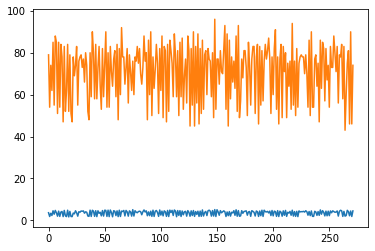In :
plt.plot(data[:, 0], data[:, 1], '.')
plt.xlabel('duration')
plt.ylabel('interval');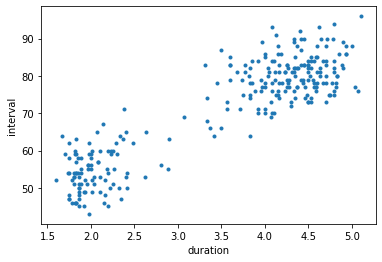We can clearly see two clusters here. For higher dimensional data, we cannot directly visualize the data to see the clusters. We need a mathematical way to detect clusters. This gives rise to the class of unsupervised learning methods called clustering algorithms.

A simple example of a clustering algorithm is the k-means algorithm. It results in identifying $k$ cluster centers. It is an iterative algorithm that starts with an initial assignment of $k$ centers. Then it proceeds by determining which centers each data sample is closest to and adjusts the centers to be the means of each of these data partitions. It then repeats.

Let's develop this algorithm one step at a time.

Each sample is the Old Faithful data has 2 attributes, so each sample is in 2-dimensional space. We know by looking at the above plot that our data nicely falls in two clusters, so we will start with $k=2$. We will initialize the two cluster centers by randomly choosing two of the data samples.

In :
data.shape

Out:
(272, 2)
In :
n_samples = data.shape
np.random.choice(range(n_samples), 2, replace=False)

Out:
array([ 28, 185])
In :
centers = data[np.random.choice(range(n_samples), 2, replace=False), :]
centers

Out:
array([[ 1.833, 63.   ],
[ 4.417, 79.   ]])

Now we must find all samples that are closest to the first center, and those that are closest to the second sample.

Check out the wonders of numpy broadcasting.

In :
a = np.array([1, 2, 3])
b = np.array([10, 20, 30])
a, b

Out:
(array([1, 2, 3]), array([10, 20, 30]))
In :
a - b

Out:
array([ -9, -18, -27])

But what if we want to subtract every element of a with every element of b?

In :
np.resize(a, (3, 3))

Out:
array([[1, 2, 3],
[1, 2, 3],
[1, 2, 3]])
In :
np.resize(b, (3, 3))

Out:
array([[10, 20, 30],
[10, 20, 30],
[10, 20, 30]])
In :
np.resize(a, (3, 3)).T

Out:
array([[1, 1, 1],
[2, 2, 2],
[3, 3, 3]])
In :
np.resize(a, (3, 3)).T - np.resize(b, (3, 3))

Out:
array([[ -9, -19, -29],
[ -8, -18, -28],
[ -7, -17, -27]])

However, we can ask numpy to do this duplication for us if we reshape a to be a column vector and leave b as a row vector.

$$\begin{pmatrix} 1\\ 2\\ 3 \end{pmatrix} - \begin{pmatrix} 10 & 20 & 30 \end{pmatrix} \;\; = \;\; \begin{pmatrix} 1 & 1 & 1\\ 2 & 2 & 2\\ 3 & 3 & 3 \end{pmatrix} - \begin{pmatrix} 10 & 20 & 30\\ 10 & 20 & 30\\ 10 & 20 & 30 \end{pmatrix}$$
In :
a

Out:
array([1, 2, 3])
In :
a[:, np.newaxis].shape

Out:
(3, 1)
In :
a[:, np.newaxis] - b

Out:
array([[ -9, -19, -29],
[ -8, -18, -28],
[ -7, -17, -27]])

Now imagine that a is a cluster center and b contains data samples, one per row. The first step of calculating the distance from a to all samples in b is to subtract them component-wise.

In :
a = np.array([1, 2, 3])
b = np.array([[10, 20, 30], [40, 50, 60]])
print(a)
print(b)

[1 2 3]
[[10 20 30]
[40 50 60]]

In :
b - a

Out:
array([[ 9, 18, 27],
[39, 48, 57]])

The single row vector a is duplicated for as many rows as there are in b! We can use this to calculate the squared distance between a center and every sample.

In :
centers[0,:]

Out:
array([ 1.833, 63.   ])
In :
sqdists_to_center_0 = np.sum((centers[0, :] - data)**2, axis=1)
sqdists_to_center_0

Out:
array([2.59122289e+02, 8.10010890e+01, 1.23250000e+02, 1.20250000e+00,
4.91290000e+02, 6.51025000e+01, 6.33219689e+02, 4.87122289e+02,
1.44013689e+02, 4.90335289e+02, 8.10000000e+01, 4.45343056e+02,
2.30602689e+02, 2.56006889e+02, 4.08219689e+02, 1.21111556e+02,
1.00688900e+00, 4.49803089e+02, 1.21054289e+02, 2.61841889e+02,
1.44001089e+02, 2.56006889e+02, 2.27614689e+02, 3.75227560e+01,
1.28290000e+02, 4.03122289e+02, 6.40179560e+01, 1.74062500e+02,
2.29068289e+02, 2.62760000e+02, 1.06086089e+02, 2.02937956e+02,
1.13531560e+01, 2.93840000e+02, 1.25000000e+02, 1.21033856e+02,
2.25001156e+02, 2.98000000e+02, 1.60000000e+01, 7.37702500e+02,
2.95335289e+02, 2.50025000e+01, 4.48474756e+02, 2.50068890e+01,
1.07290000e+02, 4.02202256e+02, 5.00000000e+00, 1.00071289e+02,
3.68840000e+02, 1.60278890e+01, 1.52803089e+02, 7.37311689e+02,
8.10000000e+01, 2.98000000e+02, 8.10100000e+01, 4.09302500e+02,
6.75494560e+01, 1.02755600e+00, 2.03474756e+02, 3.30170256e+02,
1.61600000e+01, 4.48112889e+02, 2.25006889e+02, 3.69803089e+02,
9.00025600e+00, 8.47589489e+02, 2.30447556e+02, 2.33219689e+02,
4.05475600e+00, 1.08219689e+02, 3.65840000e+02, 4.90179560e+01,
2.63112889e+02, 6.86958890e+01, 1.02250000e+00, 1.79458756e+02,
9.03385600e+00, 2.32474756e+02, 1.73202500e+02, 4.03122289e+02,
1.49290000e+02, 3.67250000e+02, 5.41392890e+01, 4.64000000e+00,
1.04990756e+02, 6.34610000e+02, 1.73481689e+02, 2.96203856e+02,
2.25111556e+02, 5.33695889e+02, 9.13468900e+00, 7.35250000e+02,
1.69001156e+02, 2.33904256e+02, 0.00000000e+00, 8.70860890e+01,
4.49031556e+02, 1.47674889e+02, 1.44001156e+02, 3.70406489e+02,
1.42250000e+00, 6.31421156e+02, 1.96071289e+02, 4.07112889e+02,
3.28915089e+02, 2.56001156e+02, 4.49219689e+02, 1.21002500e+02,
5.38102289e+02, 3.27422500e+02, 1.52410000e+02, 1.62180890e+01,
6.85406489e+02, 2.62677056e+02, 1.60176890e+01, 3.31840000e+02,
1.69234256e+02, 4.91656289e+02, 1.60002560e+01, 5.82677056e+02,
1.00614656e+02, 4.09907560e+01, 2.01841889e+02, 4.90179560e+01,
6.32656289e+02, 3.27740356e+02, 3.24007056e+02, 3.68112889e+02,
6.41883560e+01, 7.36935489e+02, 3.24001156e+02, 4.05447556e+02,
4.99350890e+01, 6.82250000e+02, 2.89000000e+02, 3.67502500e+02,
1.44002500e+02, 5.38610000e+02, 1.00040000e+02, 2.59610000e+02,
3.29760000e+02, 9.16000000e+00, 3.68290000e+02, 2.04904256e+02,
1.75250000e+02, 1.60225000e+01, 2.96840000e+02, 1.96033856e+02,
1.09967329e+03, 1.00001089e+02, 2.06240000e+02, 2.00695889e+02,
4.32148900e+00, 3.31656289e+02, 6.70067560e+01, 5.36958890e+01,
3.31112889e+02, 9.05062500e+02, 1.00001089e+02, 6.80553956e+02,
3.24134689e+02, 5.34368489e+02, 2.50278890e+01, 2.29000000e+02,
1.17788890e+01, 1.76562500e+02, 2.85156000e-01, 6.35029889e+02,
1.21010000e+02, 9.07750656e+02, 1.96007056e+02, 3.60625000e+01,
2.03562500e+02, 2.72500000e+01, 3.29447556e+02, 3.30250000e+02,
1.07112889e+02, 1.69341056e+02, 4.88695889e+02, 1.26447556e+02,
6.40025000e+01, 2.03562500e+02, 4.05841889e+02, 4.03740356e+02,
1.44040000e+02, 2.31760000e+02, 4.46062500e+02, 2.89000000e+02,
4.06677056e+02, 6.41225000e+01, 3.32803089e+02, 3.60000000e+01,
1.77803089e+02, 4.46139289e+02, 2.00549689e+02, 3.29760000e+02,
5.78778889e+02, 2.02416089e+02, 1.44173889e+02, 2.33031556e+02,
9.07128900e+00, 3.67335289e+02, 7.89290000e+02, 1.00001156e+02,
2.32656289e+02, 2.89002500e+02, 2.02421156e+02, 4.45068289e+02,
1.96010000e+02, 4.07112889e+02, 6.43025000e+01, 2.97219689e+02,
1.96001156e+02, 1.48000000e+02, 3.50905600e+00, 1.74760000e+02,
1.00321489e+02, 9.69803089e+02, 6.40278890e+01, 1.74368489e+02,
1.69001156e+02, 3.66924356e+02, 8.10068890e+01, 1.51022500e+02,
2.29695889e+02, 2.61216656e+02, 2.30062500e+02, 2.30924356e+02,
5.33430560e+01, 2.63382089e+02, 5.40625000e+01, 8.13410560e+01,
5.34522500e+02, 1.69147456e+02, 7.35848689e+02, 8.10025000e+01,
8.10002890e+01, 2.02002500e+02, 2.60481689e+02, 1.25000000e+00,
1.49368489e+02, 2.56267289e+02, 5.38610000e+02, 1.13848900e+00,
4.91562500e+02, 3.65000000e+02, 3.60625000e+01, 3.67421156e+02,
1.60900000e+01, 1.27335289e+02, 8.11346890e+01, 4.06848689e+02,
1.03006756e+02, 1.07112889e+02, 6.30368489e+02, 2.92936256e+02,
6.83430560e+01, 4.06848689e+02, 4.90278890e+01, 2.62002500e+02,
2.33608356e+02, 4.48290000e+02, 2.50002890e+01, 4.05841889e+02,
4.00022500e+02, 9.17388900e+00, 1.52508889e+02, 3.29216656e+02,
2.89100489e+02, 7.35677056e+02, 2.89000256e+02, 1.27937956e+02])
In :
sqdists_to_center_1 = np.sum((centers[1, :] - data)**2, axis=1)
sqdists_to_center_1

Out:
array([6.67489000e-01, 6.31848689e+02, 2.61750560e+01, 2.93553956e+02,
3.60134560e+01, 5.78353156e+02, 8.10800890e+01, 3.66674890e+01,
7.90086089e+02, 3.60044890e+01, 6.31677056e+02, 2.52500000e+01,
1.04708900e+00, 1.03111289e+03, 1.60800890e+01, 7.34062500e+02,
2.96112889e+02, 2.51466890e+01, 7.36935489e+02, 2.78890000e-02,
7.90848689e+02, 1.03111289e+03, 1.93508900e+00, 1.01822500e+02,
2.50134560e+01, 1.66674890e+01, 5.82002500e+02, 9.11155600e+00,
1.32148900e+00, 2.56000000e-04, 3.60136890e+01, 4.00250000e+00,
1.70102500e+02, 1.14745600e+00, 2.53410560e+01, 7.34760000e+02,
9.67502500e+02, 1.17305600e+00, 4.06677056e+02, 1.21133956e+02,
1.00448900e+00, 4.47421156e+02, 2.50225000e+01, 4.48112889e+02,
3.60134560e+01, 1.72100000e+01, 2.25341056e+02, 6.81368489e+02,
9.04665600e+00, 4.05841889e+02, 1.61466890e+01, 1.21089401e+02,
6.31677056e+02, 1.17305600e+00, 6.32203856e+02, 1.62171560e+01,
6.44900000e+01, 2.32562500e+02, 4.02250000e+00, 4.01000000e+00,
4.04769856e+02, 2.50068890e+01, 9.68112889e+02, 9.14668900e+00,
3.67760000e+02, 1.69000289e+02, 1.06250000e+00, 1.08008900e+00,
2.01522500e+02, 3.60800890e+01, 9.14745600e+00, 5.35002500e+02,
6.88900000e-03, 6.41738890e+01, 2.94924356e+02, 9.42250000e+00,
3.66760000e+02, 1.02250000e+00, 9.28515600e+00, 1.66674890e+01,
1.60806560e+01, 9.00705600e+00, 8.11004890e+01, 1.99182656e+02,
3.61225000e+01, 8.12662560e+01, 9.21808900e+00, 1.01000000e+00,
9.66062500e+02, 4.91738890e+01, 3.65915089e+02, 1.21007056e+02,
8.47502500e+02, 1.16000000e+00, 2.62677056e+02, 4.90136890e+01,
2.50625000e+01, 1.64448890e+01, 7.90502500e+02, 9.23328900e+00,
2.92740356e+02, 8.10025000e+01, 9.05368489e+02, 1.60068890e+01,
4.13468900e+00, 1.03050250e+03, 2.50800890e+01, 7.35937956e+02,
4.91874890e+01, 4.53875600e+00, 1.60998560e+01, 4.04481689e+02,
1.00233289e+02, 0.00000000e+00, 4.07382089e+02, 4.04665600e+00,
8.45410000e+02, 3.60334890e+01, 4.06760000e+02, 6.40000000e+01,
6.79240000e+02, 1.00122500e+02, 4.02788900e+00, 5.35002500e+02,
8.10334890e+01, 4.42250000e+00, 1.16225000e+03, 9.00688900e+00,
5.80622500e+02, 1.21054289e+02, 1.16250250e+03, 1.60625000e+01,
5.31614689e+02, 1.00007056e+02, 1.09567706e+03, 9.00115600e+00,
7.90421156e+02, 4.92662560e+01, 6.81683456e+02, 4.67856000e-01,
4.03385600e+00, 3.65769856e+02, 9.01345600e+00, 4.16000000e+00,
9.00705600e+00, 4.05924356e+02, 1.04665600e+00, 9.05760000e+02,
2.89466489e+02, 6.82848689e+02, 4.37945600e+00, 4.17388900e+00,
2.00068289e+02, 4.03348900e+00, 6.47225000e+01, 8.11738890e+01,
4.00688900e+00, 1.96111556e+02, 6.82848689e+02, 1.00202500e+02,
1.16091509e+03, 4.90712890e+01, 4.46841889e+02, 1.34105600e+00,
1.69840889e+02, 9.02755600e+00, 2.60202500e+02, 8.13398890e+01,
7.35170256e+02, 1.96040000e+02, 9.06250000e+02, 4.89447556e+02,
4.02755600e+00, 1.22175056e+02, 4.06250000e+00, 4.00705600e+00,
3.60068890e+01, 8.45000000e+02, 3.61738890e+01, 2.50625000e+01,
5.82421156e+02, 4.02755600e+00, 1.60278890e+01, 1.64225000e+01,
7.89683456e+02, 1.00025600e+00, 2.51115560e+01, 1.09567706e+03,
1.60000000e+01, 5.80990756e+02, 4.14668900e+00, 4.90677056e+02,
9.14668900e+00, 2.51004890e+01, 4.20340100e+00, 4.03385600e+00,
6.48408890e+01, 4.00260100e+00, 7.88695889e+02, 1.06250000e+00,
3.66368489e+02, 9.00448900e+00, 1.44080656e+02, 6.82502500e+02,
1.03348900e+00, 1.09593796e+03, 4.00250000e+00, 2.53214890e+01,
9.06170256e+02, 1.60068890e+01, 6.81371560e+01, 1.08008900e+00,
9.06502500e+02, 1.63410560e+01, 2.26000000e+02, 9.03385600e+00,
6.80068289e+02, 2.25146689e+02, 5.81841889e+02, 9.07128900e+00,
8.47502500e+02, 9.02250000e+00, 6.32112889e+02, 1.60043560e+01,
1.17388900e+00, 9.00000000e-02, 1.11155600e+00, 1.02250000e+00,
8.12500000e+01, 1.76890000e-02, 8.11115560e+01, 6.29000000e+02,
4.90547560e+01, 8.45840000e+02, 1.21001089e+02, 6.31421156e+02,
6.31589489e+02, 4.01795600e+00, 2.18089000e-01, 2.29343056e+02,
1.60712890e+01, 1.02827249e+03, 4.92662560e+01, 2.58301289e+02,
3.60275560e+01, 9.34105600e+00, 4.89447556e+02, 9.00250000e+00,
1.49216656e+02, 2.50044890e+01, 6.29915089e+02, 1.60010890e+01,
3.67225000e+01, 3.60068890e+01, 8.10712890e+01, 1.36000000e+00,
6.42500000e+01, 1.60010890e+01, 5.34841889e+02, 1.79560000e-02,
1.12250000e+00, 2.50134560e+01, 4.47589489e+02, 1.60278890e+01,
1.30192436e+03, 3.65695889e+02, 1.61108890e+01, 4.09000000e+00,
1.09413929e+03, 1.21000000e+02, 1.09576000e+03, 2.50025000e+01])

And, which samples are closest to the first center?

In :
sqdists_to_center_0 < sqdists_to_center_1

Out:
array([False,  True, False,  True, False,  True, False, False,  True,
False,  True, False, False,  True, False,  True,  True, False,
True, False,  True,  True, False,  True, False, False,  True,
False, False, False, False, False,  True, False, False,  True,
True, False,  True, False, False,  True, False,  True, False,
False,  True,  True, False,  True, False, False,  True, False,
True, False, False,  True, False, False,  True, False,  True,
False,  True, False, False, False,  True, False, False,  True,
False, False,  True, False,  True, False, False, False, False,
False,  True,  True, False, False, False, False,  True, False,
True, False,  True, False,  True, False, False, False,  True,
False,  True, False,  True, False, False,  True, False,  True,
False, False, False,  True, False, False,  True, False,  True,
False,  True, False,  True,  True, False,  True, False, False,
True, False,  True, False,  True, False,  True, False,  True,
False,  True, False,  True, False, False,  True, False, False,
False,  True, False,  True, False,  True, False, False,  True,
False, False,  True, False, False,  True, False,  True, False,
True, False,  True, False,  True, False,  True, False,  True,
True, False,  True, False, False, False,  True, False, False,
True, False, False, False,  True, False, False,  True, False,
True, False,  True, False, False, False, False, False, False,
True, False,  True, False, False,  True, False,  True, False,
False,  True, False,  True, False,  True, False,  True, False,
True, False,  True, False,  True, False,  True, False, False,
False, False, False,  True, False,  True,  True, False,  True,
False,  True,  True, False, False,  True, False,  True, False,
True, False, False,  True, False,  True, False,  True, False,
False, False, False, False, False, False,  True, False, False,
False,  True, False,  True,  True, False, False,  True, False,
True, False])

This approach is easy for $k=2$, but what if $k$ is larger. Can we calculate all of the needed distances in one numpy expression? I bet we can!

In :
centers[:,np.newaxis,:].shape, data.shape

Out:
((2, 1, 2), (272, 2))
In :
(centers[:,np.newaxis,:] - data).shape

Out:
(2, 272, 2)
In :
np.sum((centers[:,np.newaxis,:] - data)**2, axis=2).shape

Out:
(2, 272)

These are the square distances between each of our two centers and each of the 272 samples. If we take the argmin across the two rows, we will have the index of the closest center for each of the 272 samples.

In :
clusters = np.argmin(np.sum((centers[:,np.newaxis,:] - data)**2, axis=2), axis=0)
clusters

Out:
array([1, 0, 1, 0, 1, 0, 1, 1, 0, 1, 0, 1, 1, 0, 1, 0, 0, 1, 0, 1, 0, 0,
1, 0, 1, 1, 0, 1, 1, 1, 1, 1, 0, 1, 1, 0, 0, 1, 0, 1, 1, 0, 1, 0,
1, 1, 0, 0, 1, 0, 1, 1, 0, 1, 0, 1, 1, 0, 1, 1, 0, 1, 0, 1, 0, 1,
1, 1, 0, 1, 1, 0, 1, 1, 0, 1, 0, 1, 1, 1, 1, 1, 0, 0, 1, 1, 1, 1,
0, 1, 0, 1, 0, 1, 0, 1, 1, 1, 0, 1, 0, 1, 0, 1, 1, 0, 1, 0, 1, 1,
1, 0, 1, 1, 0, 1, 0, 1, 0, 1, 0, 0, 1, 0, 1, 1, 0, 1, 0, 1, 0, 1,
0, 1, 0, 1, 0, 1, 0, 1, 1, 0, 1, 1, 1, 0, 1, 0, 1, 0, 1, 1, 0, 1,
1, 0, 1, 1, 0, 1, 0, 1, 0, 1, 0, 1, 0, 1, 0, 1, 0, 0, 1, 0, 1, 1,
1, 0, 1, 1, 0, 1, 1, 1, 0, 1, 1, 0, 1, 0, 1, 0, 1, 1, 1, 1, 1, 1,
0, 1, 0, 1, 1, 0, 1, 0, 1, 1, 0, 1, 0, 1, 0, 1, 0, 1, 0, 1, 0, 1,
0, 1, 0, 1, 1, 1, 1, 1, 0, 1, 0, 0, 1, 0, 1, 0, 0, 1, 1, 0, 1, 0,
1, 0, 1, 1, 0, 1, 0, 1, 0, 1, 1, 1, 1, 1, 1, 1, 0, 1, 1, 1, 0, 1,
0, 0, 1, 1, 0, 1, 0, 1])

Now, to calculate the new values of our two centers, we just calculate the mean of the appropriate samples.

In :
data[clusters == 0, :].shape

Out:
(108, 2)
In :
centers[0,:]

Out:
array([ 1.833, 63.   ])
In :
data[clusters == 0, :].mean(axis=0)

Out:
array([ 2.20725   , 55.85185185])
In :
centers[1, :]

Out:
array([ 4.417, 79.   ])
In :
data[clusters == 1, :].mean(axis=0)

Out:
array([ 4.33106098, 80.80487805])

Can do both in a for loop.

In :
centers

Out:
array([[ 1.833, 63.   ],
[ 4.417, 79.   ]])
In :
k = 2
for i in range(k):
centers[i, :] = data[clusters == i, :].mean(axis=0)

In :
centers

Out:
array([[ 2.20725   , 55.85185185],
[ 4.33106098, 80.80487805]])

Now, we can wrap these steps in our first version of a kmeans function.

In :
def kmeans(data, k = 2, n_iterations = 5):

# Initial centers
centers = data[np.random.choice(range(data.shape), k, replace=False), :]

# Repeat n times
for iteration in range(n_iterations):

# Which center is each sample closest to?
closest = np.argmin(np.sum((centers[:, np.newaxis, :] - data)**2, axis=2),
axis=0)

# Update cluster centers
for i in range(k):
centers[i, :] = data[closest == i, :].mean(axis=0)

return centers

In :
kmeans(data, 2)

Out:
array([[ 2.09433   , 54.75      ],
[ 4.29793023, 80.28488372]])
In :
kmeans(data, 2)

Out:
array([[ 4.29793023, 80.28488372],
[ 2.09433   , 54.75      ]])

We need a measure of the quality of our clustering. For this, we define $J$, which is a performance measure being minimized by k-means. It is defined as $$J = \sum_{n=1}^N \sum_{k=1}^K r_{nk} ||\mathbf{x}_n - \mathbf{\mu}_k||^2$$ where $N$ is the number of samples, $K$ is the number of cluster centers, $\mathbf{x}_n$ is the $n^{th}$ sample and $\mathbf{\mu}_k$ is the $k^{th}$ center, each being an element of $\mathbf{R}^p$ where $p$ is the dimensionality of the data. $r_{nk}$ is 1 if $\mathbf{x}_n$ is closest to center $\mathbf{\mu}_k$, and 0 otherwise.

The sums can be computed using python for loops, but, as you know, for loops are much slower than matrix operations in python, so let's do the matrix magic. We already know how to calculate the difference between all samples and all centers.

In :
sqdists = np.sum((centers[:,np.newaxis,:] - data)**2, axis=2)
sqdists.shape

Out:
(2, 272)

The calculation of $J$ requires us to multiply the squared differences of the each component by $r_{nk}$. Since we already have all of the squared distances, let's just sum up the minimum distances for each sample.

In :
np.min(sqdists, axis=0).shape

Out:
(272,)
In :
np.sum(np.min(sqdists, axis=0))

Out:
9055.031933373459

Let's define a function named calcJ to do this calculation.

In :
def calcJ(data, centers):
sqdists = np.sum((centers[:,np.newaxis,:] - data)**2, axis=2)
return np.sum(np.min(sqdists, axis=0))

In :
calcJ(data, centers)

Out:
9055.031933373459

Now we can add this calculation to track the value of $J$ for each iteration as a kind of learning curve. $J$ measures the average "spread" within each cluster, so the smaller it is, the better.

In :
def kmeans(data, k = 2, n_iterations = 5):

# Initialize centers and list J to track performance metric
centers = data[np.random.choice(range(data.shape), k, replace=False), :]
J = []

for iteration in range(n_iterations):

# Which center is each sample closest to?
sqdistances = np.sum((centers[:, np.newaxis, :] - data)**2, axis=2)
closest = np.argmin(sqdistances, axis=0)

# Calculate J and append to list J
J.append(calcJ(data, centers))

# Update cluster centers
for i in range(k):
centers[i, :] = data[closest == i,:].mean(axis=0)

# Calculate J one final time and return results
J.append(calcJ(data, centers))
return centers, J, closest

In :
centers, J, closest = kmeans(data, 2)

In :
J

Out:
[43995.150277,
21196.418694821383,
11185.260903736931,
9020.673537301824,
8904.39799547519,
8901.76872094721]
In :
plt.plot(J);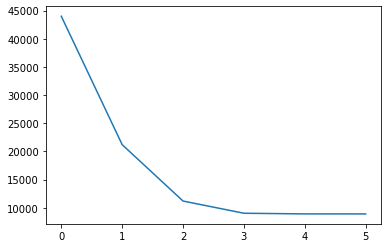In :
centers, J, closest = kmeans(data, 2, 10)
plt.plot(J)
J

Out:
[46533.820129,
11888.235099121861,
9240.152878462477,
8904.39799547519,
8901.76872094721,
8901.76872094721,
8901.76872094721,
8901.76872094721,
8901.76872094721,
8901.76872094721,
8901.76872094721]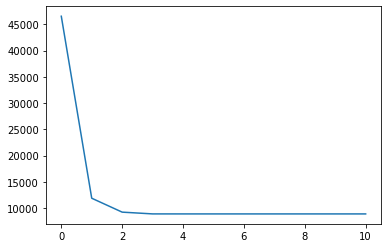In :
centers

Out:
array([[ 4.29793023, 80.28488372],
[ 2.09433   , 54.75      ]])
In :
closest

Out:
array([0, 1, 0, 1, 0, 1, 0, 0, 1, 0, 1, 0, 0, 1, 0, 1, 1, 0, 1, 0, 1, 1,
0, 0, 0, 0, 1, 0, 0, 0, 0, 0, 1, 0, 0, 1, 1, 0, 1, 0, 0, 1, 0, 1,
0, 0, 1, 1, 0, 1, 0, 0, 1, 0, 1, 0, 0, 1, 0, 0, 1, 0, 1, 0, 1, 0,
0, 0, 1, 0, 0, 1, 0, 0, 1, 0, 1, 0, 0, 0, 0, 0, 0, 1, 0, 0, 0, 0,
1, 0, 1, 0, 1, 0, 1, 0, 0, 0, 1, 0, 1, 0, 1, 0, 0, 1, 0, 1, 0, 0,
0, 1, 0, 0, 1, 0, 1, 0, 1, 0, 1, 0, 0, 1, 0, 0, 1, 0, 1, 0, 1, 0,
1, 0, 1, 0, 1, 0, 1, 0, 0, 1, 0, 0, 0, 1, 0, 1, 0, 1, 0, 0, 1, 0,
0, 0, 0, 0, 1, 0, 1, 0, 1, 0, 1, 0, 1, 0, 1, 0, 1, 1, 0, 0, 0, 0,
0, 1, 0, 0, 1, 0, 0, 0, 1, 0, 0, 1, 0, 1, 0, 1, 0, 0, 0, 0, 0, 0,
1, 0, 1, 0, 0, 1, 0, 1, 0, 0, 1, 0, 0, 0, 1, 0, 1, 0, 1, 0, 1, 0,
1, 0, 1, 0, 0, 0, 0, 0, 0, 0, 0, 1, 0, 1, 0, 1, 1, 0, 0, 1, 0, 1,
0, 1, 0, 0, 1, 0, 1, 0, 1, 0, 0, 0, 0, 0, 0, 0, 1, 0, 0, 0, 1, 0,
1, 1, 0, 0, 1, 0, 1, 0])
In :
centers

Out:
array([[ 4.29793023, 80.28488372],
[ 2.09433   , 54.75      ]])
In :
centers, J, closest = kmeans(data, 2, 2)

plt.figure(figsize=(15, 8))

plt.subplot(1, 2, 1)
plt.scatter(data[:, 0], data[:, 1], s=80, c=closest, alpha=0.5)
plt.scatter(centers[:, 0], centers[:, 1], s=80, c="green")
plt.xlabel('duration')
plt.ylabel('interval')
plt.subplot(1, 2, 2)
plt.plot(J)

centers

Out:
array([[ 3.03313333, 63.95      ],
[ 4.37731522, 84.48913043]])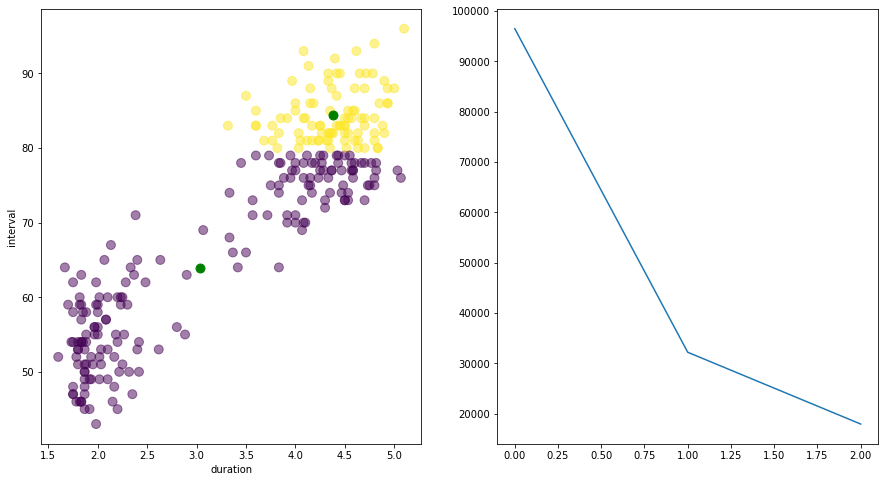In :
datast = (data - data.mean(axis=0)) / data.std(axis=0)

In :
data = datast

In :
centers, J, closest = kmeans(datast, 2, 10)

plt.figure(figsize=(15, 8))

plt.subplot(1, 2, 1)
plt.scatter(datast[:, 0], datast[:, 1], s=80, c=closest, alpha=0.5)
plt.scatter(centers[:, 0], centers[:, 1], s=80, c="green")

plt.subplot(1, 2, 2)
plt.plot(J)

centers

Out:
array([[ 0.70970327,  0.67674488],
[-1.26008539, -1.20156744]])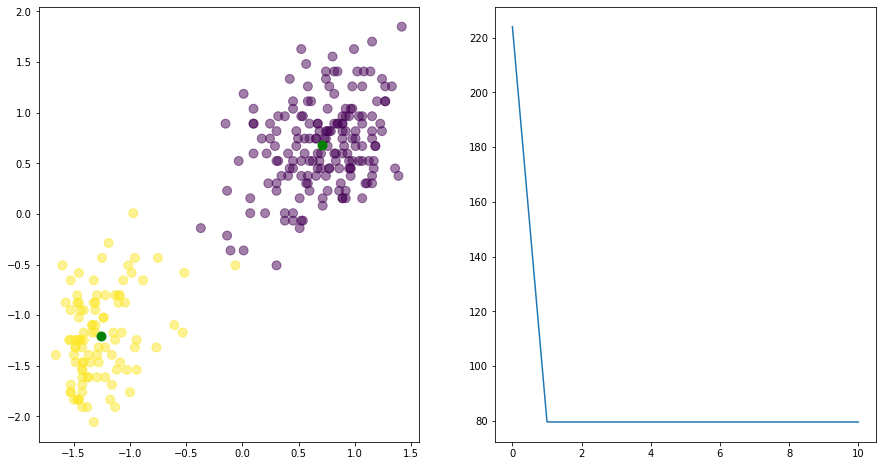In :
centers, J, closest = kmeans(data, 3, 10)

plt.figure(figsize=(15, 8))

plt.subplot(1, 2, 1)
plt.scatter(data[:, 0], data[:, 1], s=80, c=closest, alpha=0.5)
plt.scatter(centers[:, 0], centers[:, 1], s=80, c="green")

plt.subplot(1, 2, 2)
plt.plot(J)

centers

Out:
array([[-1.04885744, -0.69064749],
[-1.32475114, -1.47101207],
[ 0.72993452,  0.69899205]])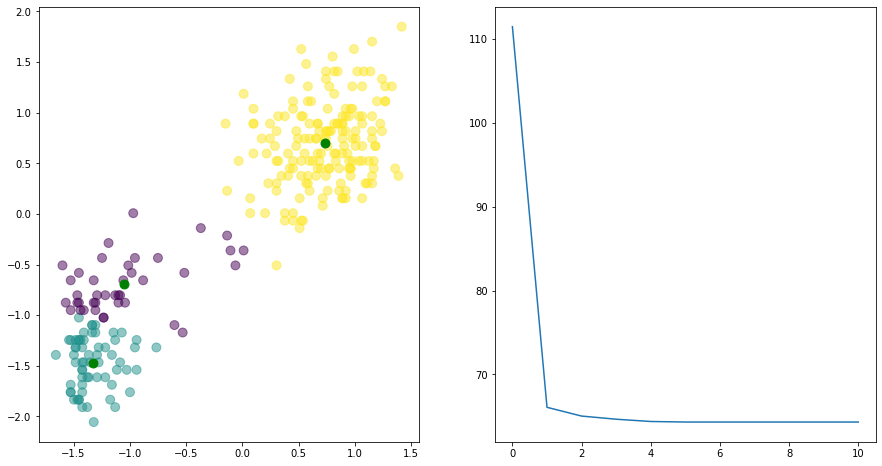In :
centers, J, closest = kmeans(data, 4, 10)

plt.figure(figsize=(15, 8))

plt.subplot(1, 2, 1)
plt.scatter(data[:, 0], data[:, 1], s=80, c=closest, alpha=0.5)
plt.scatter(centers[:, 0], centers[:, 1], s=80, c="green")

plt.subplot(1, 2, 2)
plt.plot(J)

centers

Out:
array([[ 0.89401996,  0.90031414],
[ 0.42220204,  0.32446235],
[-1.19357662, -0.79763596],
[-1.33017137, -1.50968349]])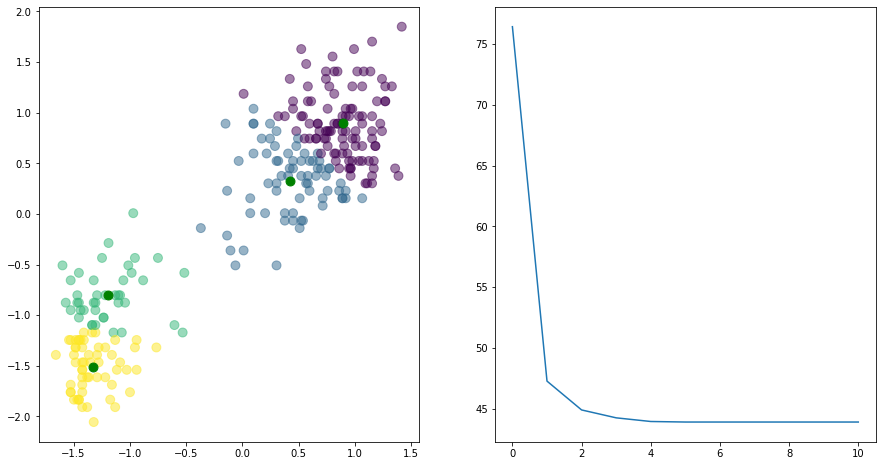In :
centers, J, closest = kmeans(data, 6, 20)

plt.figure(figsize=(15, 8))

plt.subplot(1, 2, 1)
plt.scatter(data[:, 0], data[:, 1], s=80, c=closest, alpha=0.5)
plt.scatter(centers[:, 0], centers[:, 1], s=80, c="green")

plt.subplot(1, 2, 2)
plt.plot(J)

centers

Out:
array([[ 0.38668748,  0.77582699],
[-1.33017137, -1.50968349],
[ 0.30534616,  0.03807928],
[ 0.92647178,  0.56659382],
[-1.19357662, -0.79763596],
[ 0.95140766,  1.26240011]])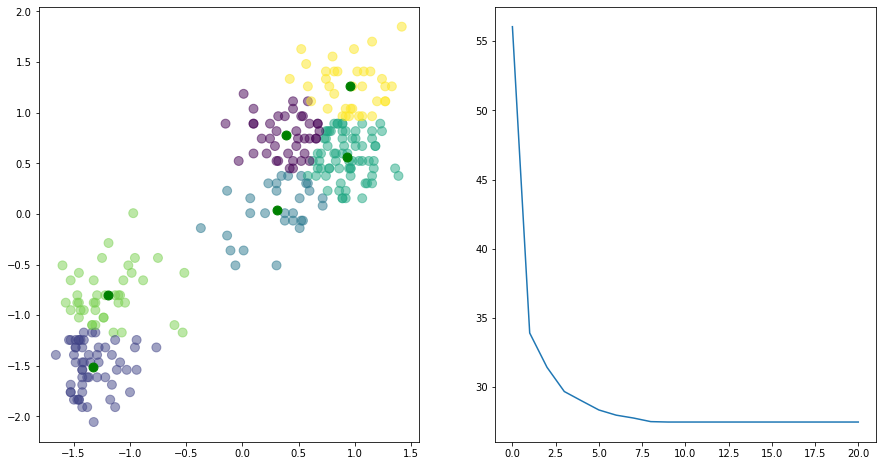## MNIST Dataset¶

So, clustering two-dimensional data is not all that exciting. How about 784-dimensional data, such as our good buddy the MNIST data set?

In :
import gzip
import pickle

with gzip.open('mnist.pkl.gz', 'rb') as f:
train_set, valid_set, test_set = pickle.load(f, encoding='latin1')

Xtrain = train_set
Ttrain = train_set.reshape((-1,1))

Xtest = test_set
Ttest = test_set.reshape((-1,1))

Xtrain.shape, Ttrain.shape, Xtest.shape, Ttest.shape

Out:
((50000, 784), (50000, 1), (10000, 784), (10000, 1))

How many clusters shall we use?

In :
centers, J, closest = kmeans(Xtrain, k=2, n_iterations=10)

In :
plt.plot(J)
J

Out:
[5604641.5,
2521244.0,
2489575.5,
2476870.0,
2470153.2,
2466284.0,
2463962.2,
2462255.0,
2460865.8,
2459661.2,
2458532.5]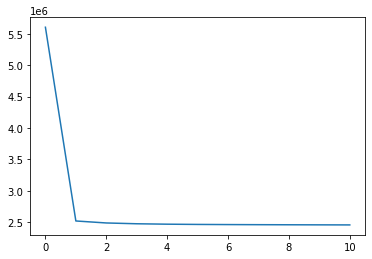In :
centers.shape

Out:
(2, 784)
In :
centers, J, closest = kmeans(Xtrain, k=3, n_iterations=10)

for i in range(3):
plt.subplot(2, 5, i + 1)
plt.imshow(-centers[i, :].reshape((28, 28)), cmap='gray')
plt.axis('off')In :
centers, J, closest = kmeans(Xtrain, k=10, n_iterations=20)
plt.plot(J)
plt.figure()
for i in range(10):
plt.subplot(2, 5, i + 1)
plt.imshow(-centers[i, :].reshape((28, 28)), cmap='gray')
plt.axis('off')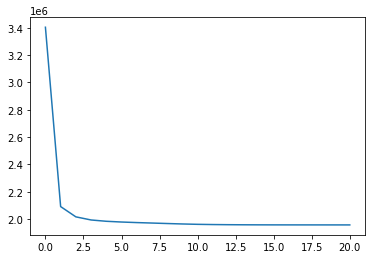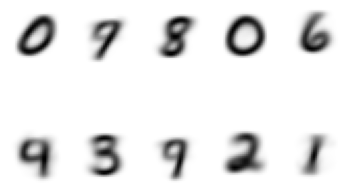In :
centers, J, closest = kmeans(Xtrain, k=20, n_iterations=20)
plt.plot(J)
plt.figure()
for i in range(20):
plt.subplot(4, 5, i + 1)
plt.imshow(-centers[i, :].reshape((28, 28)), interpolation='nearest', cmap='gray')
plt.axis('off')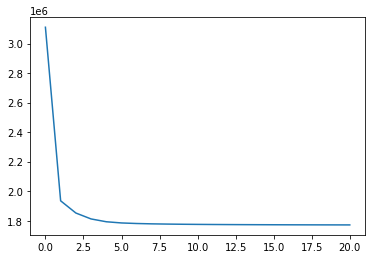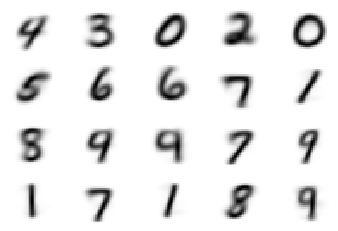In :
centers, J, closest = kmeans(Xtrain, k=20, n_iterations=20)
plt.plot(J)
plt.figure()
for i in range(20):
plt.subplot(4, 5, i + 1)
plt.imshow(-centers[i, :].reshape((28, 28)), interpolation='nearest', cmap='gray')
plt.axis('off')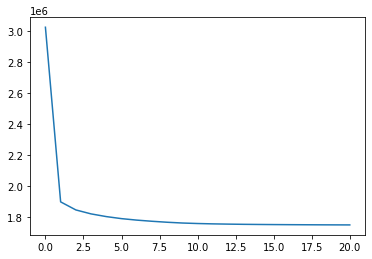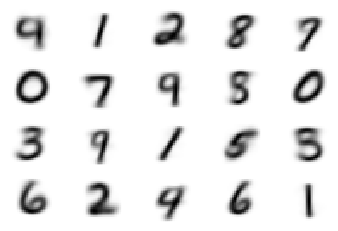In :
centers, J, closest = kmeans(Xtrain, k=40, n_iterations=20)
plt.plot(J)
plt.figure()
for i in range(40):
plt.subplot(4, 10, i + 1)
plt.imshow(-centers[i, :].reshape((28, 28)), interpolation='nearest', cmap='gray')
plt.axis('off')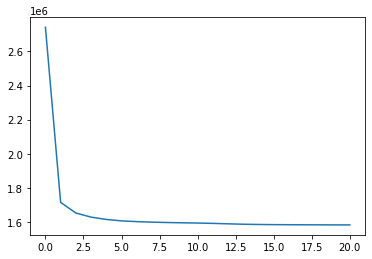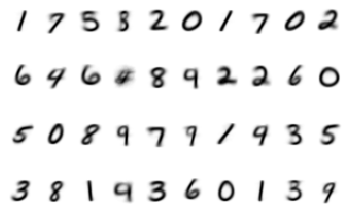How could you use the results of the kmeans clustering algorithm as the first step in a classification algorithm?

# Clustering of Word Embeddings¶

In :
import transformers as tr

# initialize the model architecture and weights
model = tr.T5ForConditionalGeneration.from_pretrained("t5-base")
# initialize the model tokenizer
tokenizer = tr.T5Tokenizer.from_pretrained("t5-base")

In :
text = """
Julia was designed from the beginning for high performance. Julia programs compile to efficient
native code for multiple platforms via LLVM.
Julia is dynamically typed, feels like a scripting language, and has good support for interactive use.
Reproducible environments make it possible to recreate the same Julia environment every time,
across platforms, with pre-built binaries.
Julia uses multiple dispatch as a paradigm, making it easy to express many object-oriented
and functional programming patterns. The talk on the Unreasonable Effectiveness of Multiple
Dispatch explains why it works so well.
Julia provides asynchronous I/O, metaprogramming, debugging, logging, profiling, a package manager,
and more. One can build entire Applications and Microservices in Julia.
Julia is an open source project with over 1,000 contributors. It is made available under the
MIT license. The source code is available on GitHub.
"""

In :
inputs = tokenizer.encode("summarize: " + text, return_tensors="pt", truncation=True)

In :
tokenizer

Out:
PreTrainedTokenizer(name_or_path='t5-base', vocab_size=32100, model_max_len=512, is_fast=False, padding_side='right', special_tokens={'eos_token': '</s>', 'unk_token': '<unk>', 'pad_token': '<pad>', 'additional_special_tokens': ['<extra_id_0>', '<extra_id_1>', '<extra_id_2>', '<extra_id_3>', '<extra_id_4>', '<extra_id_5>', '<extra_id_6>', '<extra_id_7>', '<extra_id_8>', '<extra_id_9>', '<extra_id_10>', '<extra_id_11>', '<extra_id_12>', '<extra_id_13>', '<extra_id_14>', '<extra_id_15>', '<extra_id_16>', '<extra_id_17>', '<extra_id_18>', '<extra_id_19>', '<extra_id_20>', '<extra_id_21>', '<extra_id_22>', '<extra_id_23>', '<extra_id_24>', '<extra_id_25>', '<extra_id_26>', '<extra_id_27>', '<extra_id_28>', '<extra_id_29>', '<extra_id_30>', '<extra_id_31>', '<extra_id_32>', '<extra_id_33>', '<extra_id_34>', '<extra_id_35>', '<extra_id_36>', '<extra_id_37>', '<extra_id_38>', '<extra_id_39>', '<extra_id_40>', '<extra_id_41>', '<extra_id_42>', '<extra_id_43>', '<extra_id_44>', '<extra_id_45>', '<extra_id_46>', '<extra_id_47>', '<extra_id_48>', '<extra_id_49>', '<extra_id_50>', '<extra_id_51>', '<extra_id_52>', '<extra_id_53>', '<extra_id_54>', '<extra_id_55>', '<extra_id_56>', '<extra_id_57>', '<extra_id_58>', '<extra_id_59>', '<extra_id_60>', '<extra_id_61>', '<extra_id_62>', '<extra_id_63>', '<extra_id_64>', '<extra_id_65>', '<extra_id_66>', '<extra_id_67>', '<extra_id_68>', '<extra_id_69>', '<extra_id_70>', '<extra_id_71>', '<extra_id_72>', '<extra_id_73>', '<extra_id_74>', '<extra_id_75>', '<extra_id_76>', '<extra_id_77>', '<extra_id_78>', '<extra_id_79>', '<extra_id_80>', '<extra_id_81>', '<extra_id_82>', '<extra_id_83>', '<extra_id_84>', '<extra_id_85>', '<extra_id_86>', '<extra_id_87>', '<extra_id_88>', '<extra_id_89>', '<extra_id_90>', '<extra_id_91>', '<extra_id_92>', '<extra_id_93>', '<extra_id_94>', '<extra_id_95>', '<extra_id_96>', '<extra_id_97>', '<extra_id_98>', '<extra_id_99>']})
In :
inputs.size()

Out:
torch.Size([1, 199])
In :
outputs = model.generate(inputs, max_length=100, min_length=10, length_penalty=1.0, num_beams=4,
num_return_sequences=1)

In :
tokenizer.decode(outputs)

Out:
'Julia is dynamically typed, feels like a scripting language, and has good support for interactive use. Julia provides asynchronous I/O, metaprogramming, debugging, logging, profiling, a package manager, and more. the source code is available on GitHub under the MIT license.'
In :
embedding = model.encoder.embed_tokens(inputs)
embedding.shape

Out:
torch.Size([1, 199, 768])
In :
embeddings = model.encoder.embed_tokens(inputs)
embeddings

Out:
tensor([[[ 31.5000,  15.3125,  11.9375,  ...,   7.2188, -13.2500,  -8.3750],
[ -8.0000,  -2.5938,  -0.7070,  ...,   0.5391,  -7.2188,  21.8750],
[-14.5625,   4.4688, -11.2500,  ...,   3.7656, -17.2500,   6.5938],
...,
[ 16.7500,   1.3125, -25.7500,  ...,  -5.2812,  -6.3125,  -1.4062],
[ 13.5000,  -5.5938,   8.6250,  ...,   8.9375,   7.9688,  -5.5625],
[ 11.3750,  -4.8750,   9.0625,  ...,   4.8438,  14.3750,  -5.7812]]],
grad_fn=<EmbeddingBackward>)
In :
embeddings.shape

Out:
torch.Size([1, 199, 768])
In :
embeddings = embeddings[0, ...]

In :
embeddings.shape

Out:
torch.Size([199, 768])
In :
embeddingsnp = embeddings.detach().numpy()

In :
centers, J, closest = kmeans(embeddingsnp, 10, 1000)

In :
np.unique(closest, return_counts=True)

Out:
(array([0, 1, 2, 3, 4, 5, 6, 7, 8, 9]),
array([ 1,  1, 80,  1,  1,  1, 22,  6, 83,  3]))
In :
tokenizer.decode(inputs[0, closest==7])

Out:
'designed makebuilt making build made'
In :
tokenizer.decode(inputs[0, closest==9])

Out:
'programs programmingprogramm'
In [ ]: